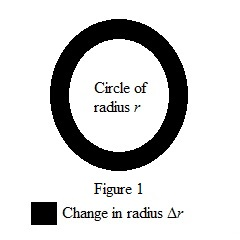# The average rate of change of the area of a circle with respect to its radius r as r change from 2 to 3.### Single Variable Calculus: Concepts...

4th Edition
James Stewart
Publisher: Cengage Learning
ISBN: 9781337687805### Single Variable Calculus: Concepts...

4th Edition
James Stewart
Publisher: Cengage Learning
ISBN: 9781337687805

#### Solutions

Chapter 3.8, Problem 13E

(a)

(i)

To determine

## The average rate of change of the area of a circle with respect to its radius r as r change from 2 to 3.

Expert Solution

The average rate of change of the area of a circle is 5π_.

### Explanation of Solution

Expression for the area of the circle is given as below.

A(r)=πr2

Calculate the average rate of change of the area of a circle, when the radius changes from 2 to 3.

A(r)=A(3)A(2)32A(r)=9π4π1A(r)=5π

Therefore, the average rate of change of the area of a circle is 5π_.

(ii)

To determine

### The average rate of change of the area of a circle with respect to its radius r as r change from 2 to 2.5.

Expert Solution

The average rate of change of the area of a circle is 4.5π_.

### Explanation of Solution

Calculate the average rate of change of the area of a circle, when the radius changes from 2 to 2.5.

A(r)=A(2.5)A(2)2.52A(r)=6.25π4π0.5A(r)=4.5π

Therefore, the average rate of change of the area of a circle is 4.5π_.

(iii)

To determine

### The average rate of change of the area of a circle with respect to its radius r as r change from 2 to 2.1.

Expert Solution

The average rate of change of the area of a circle is 4.1π_

### Explanation of Solution

Calculate the average rate of change of the area of a circle, when the radius changes from 2 to 2.1.

A(r)=A(2.1)A(2)2.12A(r)=4.41π4π0.1A(r)=4.1π

Therefore, the average rate of change of the area of a circle is 4.1π_.

b)

To determine

### The instantaneous rate of change when r = 2.

Expert Solution

When r is 2 the instantaneous rate of change is 4π_.

### Explanation of Solution

Calculate the instantaneous rate of change when r is 2 using the below equation.

A(r)=πr2

Differentiate the above area equation,

A'(r)=2πr (1)

Substitute the value 2 for r.

A'(2)=2π(2)A'(2)=4π

Therefore, when the value of r is 2 the instantaneous rate of change is 4π_.

c)

To determine

### To show: The rate of change of the area of a circle with respect to its radius is equal to the circumference of the circle and why it is geometrically true.

Expert Solution

It is geometrically true. Hence, the resulting change in area ΔA if change in radius Δr is small is ΔAΔr2πr.

### Explanation of Solution

Show that the rate of change of the area of a circle with respect to its radius is equal to the circumference of the circle.

Circumference of the circle is given as below.

c(r)=2πr (2)

First derivative of the area of the circle is given as below from the equation (1).

A'(r)=2πr

Compare both the equations (1) and (2).

c(r)=A'(r)=2πr

Consider a circular ring of radius r and strip of thickness Δr along the radial direction representing the change in the radius for the circle as shown in figure (1).Show that if the value of Δr is small, then the change in the circle is approximately equal to its circumference.

Explain why it is geometrically true.

Approximate the resulting change in area ΔA, if the value of Δr is very small.

Write the expression for ΔA in algebraically manner.